Pull Down & Release to Refresh

# Year 10+ Coordinate Geometry

### Chapters

$ax^2 + bx + c = 0$
There are three different constants in a quadratic equation: $a$, $b$ and $c$. You can set them equal to anything you like. The only condition is that $a$ cannot be zero, or you'll just end up with a straight line.

We are interested in solving quadratic equations. To do this, it helps to graph the function

$y = ax^2 + bx + c$
and check if and where it crosses the $x$-axis. The $x$-intercepts correspond to solutions of the quadratic equation.

## The Function $y = ax^2$

The simplest quadratic function is $y = ax^2$. Its graph is a parabola, with its vertex at $(0,0)$. Let's see how the graph changes for different values of $a$.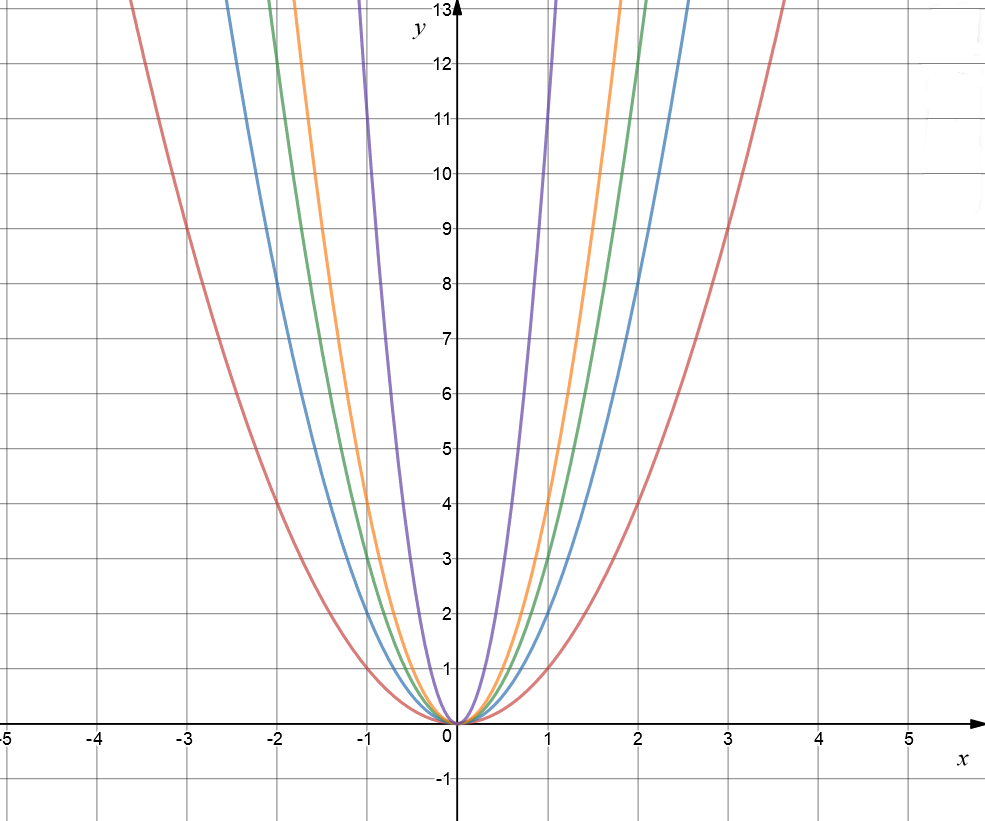Let's see what happens to graph if we set $b = c = 0$, and $a$ to different positive values. In the picture, the red graph shows $y = x^2$, the blue graph is $y = 2x^2$, the green graph is $y = 3x^2$, and the orange graph is $y = 4x^2$. I went all out for the purple graph: it is $y = 11x^2$. Do you notice how, as we increase the value of $a$, the graph gets steeper and narrower? The graph gets wider and slopes more gradually as we decrease the value of $a$.

### Everything's Topsy-Turvy with Negative Values of $a$

If we make $a$ negative, we turn the graph upside down.

The red graph is $y = -2x^2 + 3x + 4$, the black graph is $y = -x^2 + 3x$, and the green graph is $y = -x^2$. They are all upside down parabolas.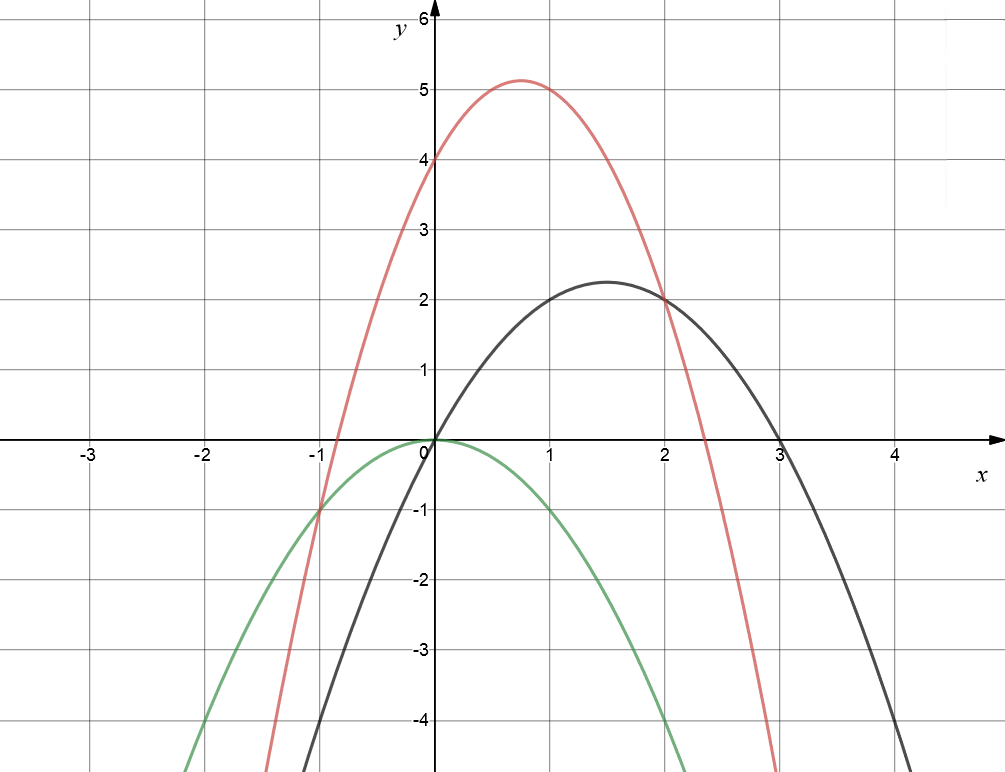The standard form of the general quadratic function is $f(x) = ax^2 + bx + c$. When we need to graph a quadratic function, there's another form that can help us out. It's called the vertex form of the quadratic function. The vertex is the minimum point (if the parabola is right way up) or the maximum point (if the parabola is upside down) of the parabola. The vertex form of the quadratic function looks like this:

$f(x) = a(x - h)^2 + k$
To turn the first equation into the second, we don't need any special magic. We just need to know that
• $h = - \dfrac{b}{2a}$ and
• $k = f(h)$
then the vertex of the parabola will be at the point $(h,k)$. So, in other words, to convert a standard form equation into vertex form we:
1. Calculate $h = -\dfrac{b}{2a}$ and then
2. Plug our new value of $h$, which is the $x$-coordinate of the vertex, into the equation for $f(x)$ to find the $y$-coordinate of the vertex.

When the equation is in vertex form, we can not only read off the coordinates of the vertex, but we also know the equation of the axis of symmetry of the parabola. It's the line $x = h$. The graph is symmetric about this line.

• The value of $h$ tells us how far to the left or right the graph of $y = ax^2$ has been shifted, and
• the value of $k$ tells us how far up or down the graph of $y = ax^2$ has been shifted
to form the graph of $y = ax^2 + bx + x = a(x - h)^2 + k$.

Let's see how this appears on the graph: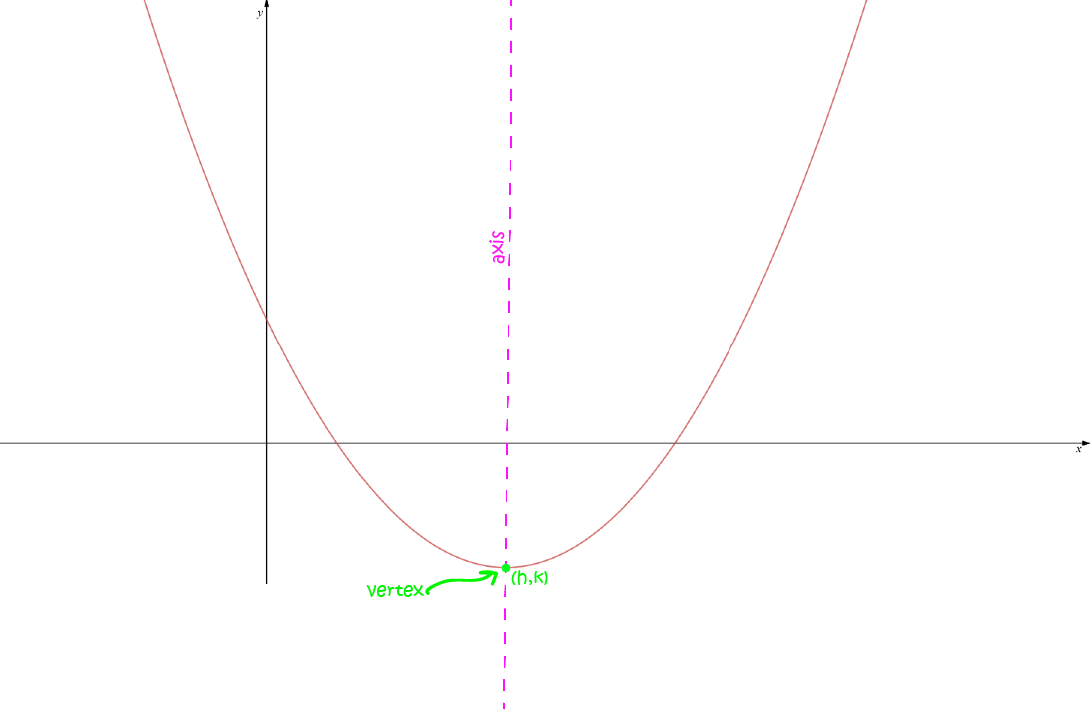Let's work through an example.

### Example

Find the graph of $f(x) = 3x^2 - 18x + 15$.

Solution: In this equation

• $a = 3$
• $b = -18$
• $c = 15$

Because $a > 0$, the parabola faces upwards (it is U-shaped).

Because $a = 3$, the parabola is thinner than the graph of $y = x^2$.

Next, find $h$ and $k$:

$h = - \dfrac{b}{2a} = - \dfrac{-18}{2 \times 3} = \dfrac{18}{6} = 3$
Use the equation for $f(x)$ to find $k$:
$k = f(3) = 3(3)^2 - 18 (3) + 15 = 27 - 54 + 15 = -12$
and we can plot the graph.

Step 1: Start with $y = x^2$: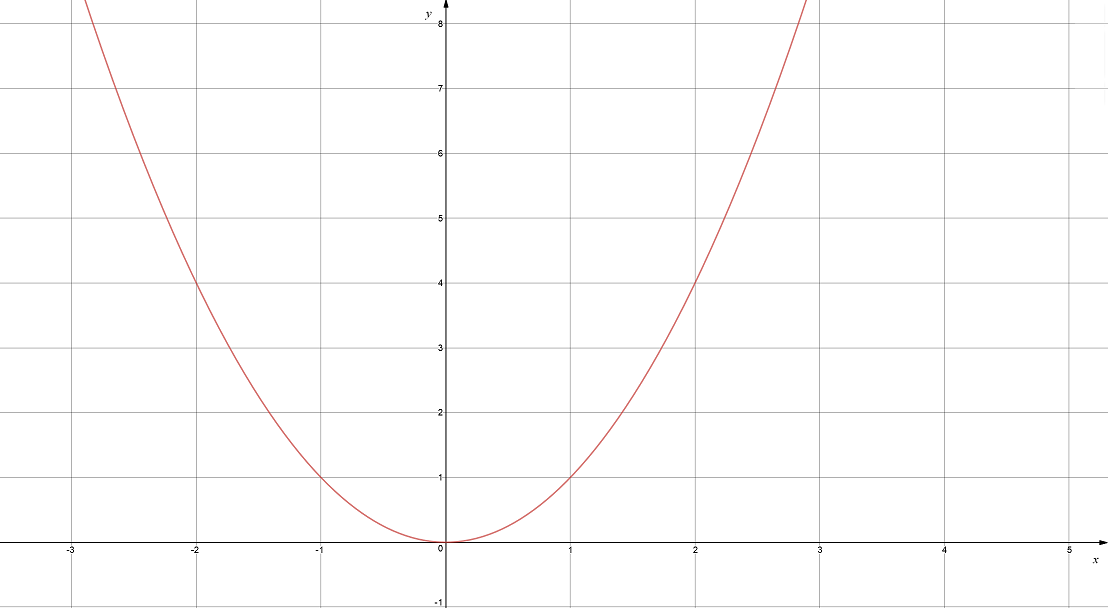Step2: Make it skinny to form $y = 3x^2$: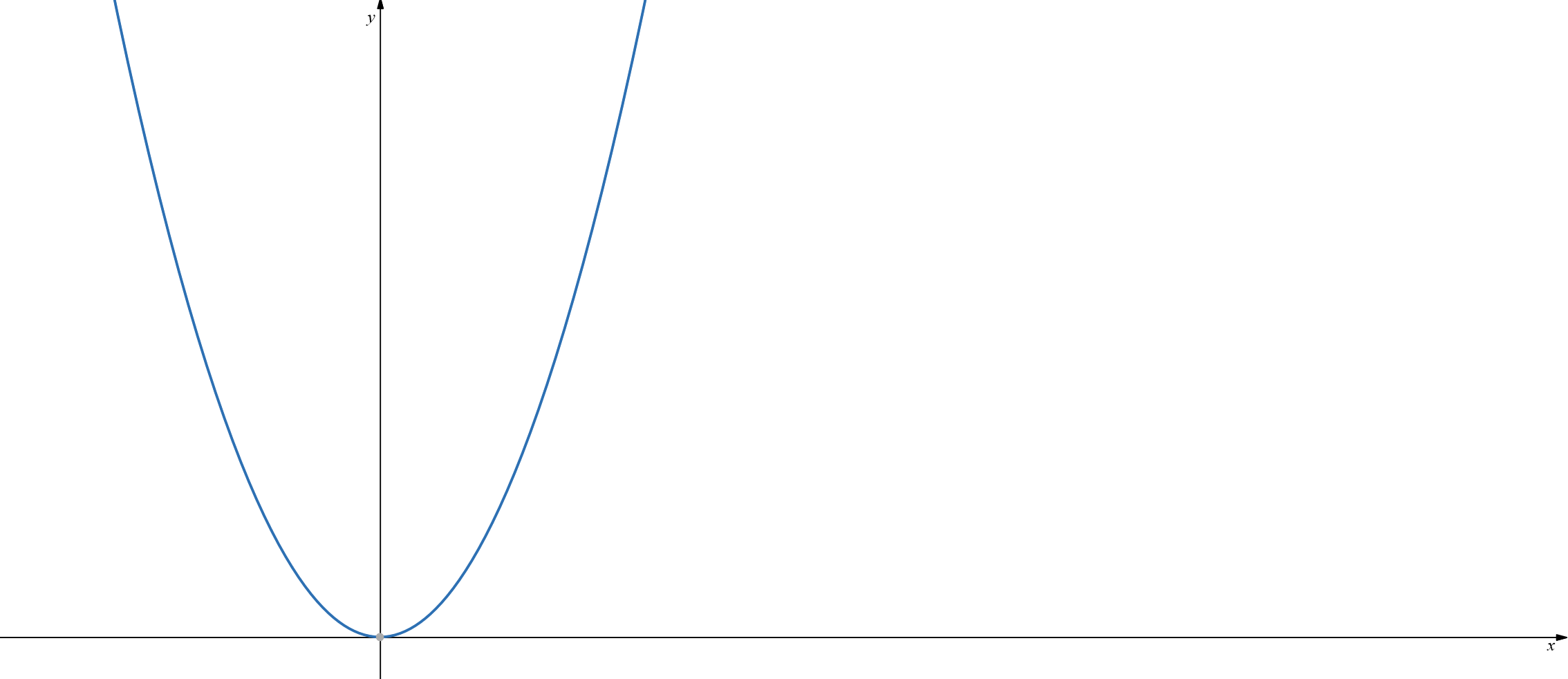Step3: Move the graph across and down so that the vertex lands at $(3,-12)$: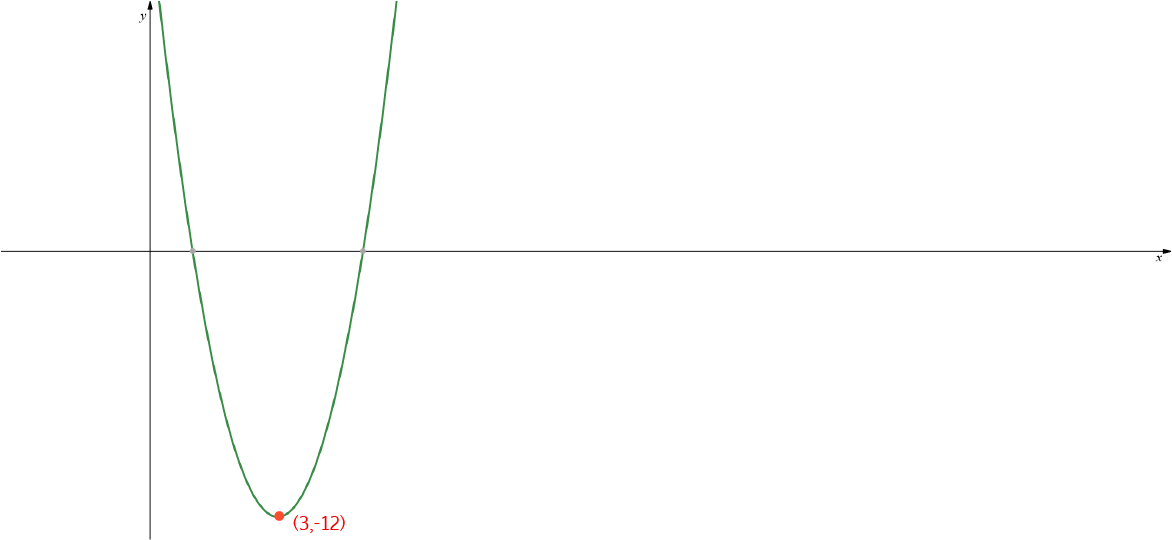The vertex is at $(3,-12)$, and the axis is the line $x = 3$.

## Can You Find the Equation if You Know What the Graph Looks Like?

Let's look at an example.

### Example:

Suppose you have plotted some points from a data set, and it looks like this: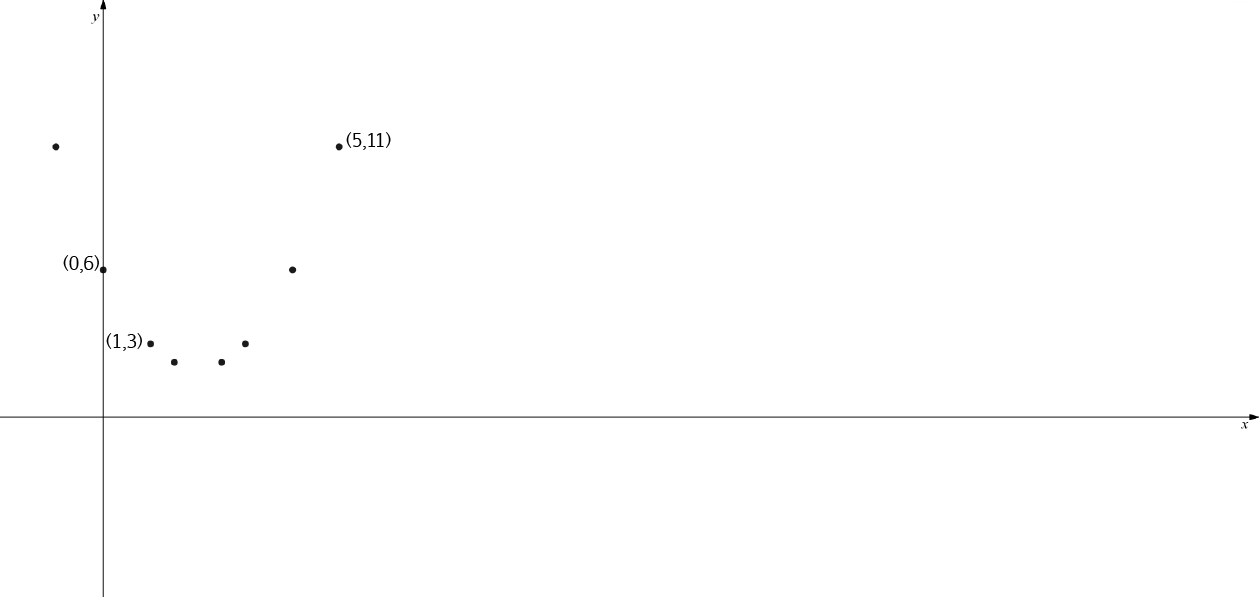It looks a bit like a parabola, so it's a good idea to use the equation of a parabola to predict other data values. It may not be completely accurate, but it will give a good approximation.

To find the equation of a parabola, we only need to know three points. In this example, we know the points $(0,6)$, $(1,3)$ and $(5,11)$. We can plug these into the standard form of the equation of a parabola to find the values of $a$, $b$ and $c$, and hence the equation of the parabola.

• Using the point $(0,6)$, we obtain $6 = a(0)^2 + b(0) + c$, so $c = 6$.
• Using the point $(1,3)$, we obtain $3 = a(1)^2 + b(1) + 6$, so $a + b = -3$.
• Finally using the point $(5,11)$, we obtain $11 = a(5)^2 + b(5) + 6$, so $25 a + 5b = 5$, and dividing by $5$, $5a + b = 1$.
• We can solve the last two equations simultaneously to find the values of $a$ and $b$, which are $a = 1$, and $b = -4$.

If you can't remember how to solve equations simultaneously, it would be a good idea to read the articles on solving simultaneous equations.

Finally, the equation of our quadratic function is

$f(x) = x^2 - 4x + 6$

Why not have a play around with some more quadratic equations, yourself, and then check your results using an on-line graphing calculator? Have fun!

### Description

coordinate geometry is a branch of geometry where the position of the points on the plane is defined with the help of an ordered pair of numbers also known as coordinates. In this tutorial series, you will learn about vast range of topics such as Cartesian Coordinates, Midpoint of a Line Segment etc

### Audience

year 10 or higher, several chapters suitable for Year 8+ students.

### Learning Objectives

Explore topics related to Coordinates Geometry

Author: Subject Coach
You must be logged in as Student to ask a Question.